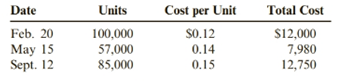Chapter 6, Problem 70BPSB### Cornerstones of Financial Accounti...

4th Edition
Jay Rich + 1 other
ISBN: 9781337690881

#### Solutions

Chapter
Section### Cornerstones of Financial Accounti...

4th Edition
Jay Rich + 1 other
ISBN: 9781337690881
Textbook Problem
1 views

# Inventory Costing and LCMJ&J Enterprises sells paper cups to fast-food franchises. On January 1, 2019, J&J had 5,000 cups on hand, for which it had paid $0.10 per cup. During 2019, J&J made the following purchases and sales:During 2019, J&J sold 240,000 cups at$0.35 per cup (80,000 cups were sold on April 2 and 160,000cups were sold on October 20), leaving an ending inventory of 7,000 cups. Assume that J&J uses a perpetual inventory system. J&J uses the lower of cost or market for its inventories, as required by generally accepted accounting principles.Required:1. Assume that the market value of the cups is $0.38 per cup on December 31, 2019. Compute the cost of ending inventory using the FIFO and average cost methods, and then apply LCM. ( Note: Use four decimal places for per-unit calculations and round all other numbers to the nearest dollar.)2. Assume that the market value of the cups is$0.12 per cup on December 31, 2019. Compute the cost of ending inventory using the FIFO and average cost methods, and then apply LCM. ( Note: Use four decimal places for per-unit calculations and round all other numbers to the nearest dollar.)

To determine

(a)

Introduction:

The first in, first out (FIFO) method of inventory valuation is a cost stream supposition that the first products obtained are likewise the first merchandise sold. In many organizations, this presumption intently coordinates the genuine stream of merchandise, as is viewed as the most hypothetically right inventory valuation method.

The average cost method is an inventory costing method in which the cost of every thing in an inventory is determined on the basis of the average cost of every comparative great in the inventory. The average cost method is determined by partitioning the cost of products in inventory by the absolute number of things accessible available to be purchased.

To compute:

Assume that the market value of the cups is $0.38 per cup on December 31, 2019. Compute the cost of ending inventory using the FIFO and average cost methods, and then apply LCM. Explanation In this method, first in first out means first received stock firstly sold. Closing inventory 7000 units is only out of 85000 units Value of closing stock = 7000 units × 0.15 = 1050 (Market value 0.38 or cost 0.15 whichever is lower) In this method, average cost method Calculate average cost = 0 To determine (b) Introduction: The first in, first out (FIFO) method of inventory valuation is a cost stream supposition that the first products obtained are likewise the first merchandise sold. In many organizations, this presumption intently coordinates the genuine stream of merchandise, as is viewed as the most hypothetically right inventory valuation method. The average cost method is an inventory costing method in which the cost of every thing in an inventory is determined on the basis of the average cost of every comparative great in the inventory. The average cost method is determined by partitioning the cost of products in inventory by the absolute number of things accessible available to be purchased. To compute: Assume that the market value of the cups is$0.12 per cup on December 31, 2019. Compute the cost of ending inventory using the FIFO and average cost methods, and then apply LCM.

### Still sussing out bartleby?

Check out a sample textbook solution.

See a sample solution

#### The Solution to Your Study Problems

Bartleby provides explanations to thousands of textbook problems written by our experts, many with advanced degrees!

Get Started

#### Why is understanding motivation important?

Foundations of Business (MindTap Course List)

#### Give examples of practices that you need to follow to be successful in college.

Engineering Fundamentals: An Introduction to Engineering (MindTap Course List)

#### Briefly explain the purpose of a sampling plan.

Precision Machining Technology (MindTap Course List)